# JP4476049B2 - Brushless motor control device - Google Patents

## Info

Publication number
JP4476049B2
JP4476049B2 JP2004194390A JP2004194390A JP4476049B2 JP 4476049 B2 JP4476049 B2 JP 4476049B2 JP 2004194390 A JP2004194390 A JP 2004194390A JP 2004194390 A JP2004194390 A JP 2004194390A JP 4476049 B2 JP4476049 B2 JP 4476049B2
Authority
JP
Japan
Prior art keywords
boost
value
control
motor
means
Prior art date
Legal status (The legal status is an assumption and is not a legal conclusion. Google has not performed a legal analysis and makes no representation as to the accuracy of the status listed.)
Expired - Fee Related
Application number
JP2004194390A
Other languages
Japanese (ja)
Other versions
JP2006020399A (en
Inventor

Original Assignee

Priority date (The priority date is an assumption and is not a legal conclusion. Google has not performed a legal analysis and makes no representation as to the accuracy of the date listed.)
Filing date
Publication date
Application filed by 三洋電機株式会社 filed Critical 三洋電機株式会社
Priority to JP2004194390A priority Critical patent/JP4476049B2/en
Publication of JP2006020399A publication Critical patent/JP2006020399A/en
Application granted granted Critical
Publication of JP4476049B2 publication Critical patent/JP4476049B2/en
Application status is Expired - Fee Related legal-status Critical
Anticipated expiration legal-status Critical

## Images

•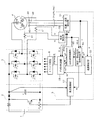•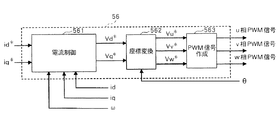•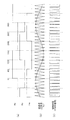•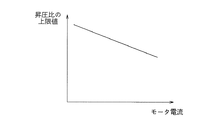•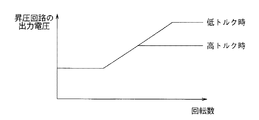•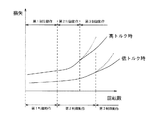•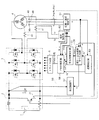•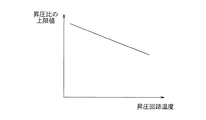•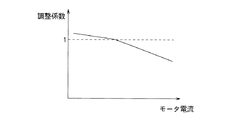•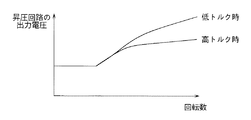•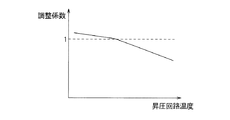•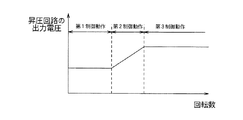•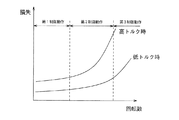## Description

The present invention relates to a brushless motor control device including a booster circuit and an inverter that converts an output voltage of the booster circuit into an alternating current and supplies the same to a brushless motor.

2. Description of the Related Art Conventionally, there has been known a motor control device that includes a booster circuit to increase the maximum number of rotations of a brushless motor, perform boosting control, and perform field weakening control to reduce magnetic flux generated in the motor (see, for example, Patent Document 1). ).
As shown in FIG. 12, this type of motor control device first executes a first control operation in which PWM control is performed without performing step-up control and field weakening control, and then the rotational speed of the motor reaches a predetermined rotational speed. At this point, the second control operation for performing boost control is started. Furthermore, when the boost ratio of the booster circuit reaches the upper limit value, a third control operation for performing boost control and field weakening control is started.
In this way, the maximum number of revolutions of the motor can be increased by performing boost control and field weakening control.
JP 2003-199382 A

However, according to the applicant's research, it has been found that in the conventional motor control device, the temperature rise of the booster circuit may be large.
Accordingly, an object of the present invention is to provide a brushless motor control device capable of preventing an increase in temperature of a booster circuit.

The applicant has investigated the cause of the increase in the temperature of the booster circuit as follows.
FIG. 13 shows a change in the magnitude of loss that occurs when the motor is driven. As shown in the figure, the loss increases slowly when the motor rotation speed is small, and increases rapidly when the motor rotation speed increases. However, when the torque is high, the loss rapidly increases at a smaller rotational speed than when the torque is low.
In the conventional motor control device, the upper limit value of the step-up ratio is set to a large value at the time when the loss suddenly increases at low torque, so the point at which the loss increases rapidly at low torque Although the magnetic flux weakening control is started and the temperature rise of the booster circuit is suppressed to a small value, at high torque, the magnetic flux weakening control is started after the loss increases rapidly, and the temperature rise of the booster circuit is large. Become.

A brushless motor control device according to the present invention includes a booster circuit that boosts a DC input voltage, an inverter that converts an output voltage of the booster circuit into AC and supplies the brushless motor, a boost ratio of the booster circuit, and an operation of the inverter And a control circuit that controls the operation of the booster circuit after the boost control operation for setting the boost ratio of the booster circuit to a value larger than 1 is started, and an operation for reducing the magnetic flux generated from the brushless motor The magnetic flux control operation to be executed is started. In the characteristic configuration, the control circuit changes the time point at which the magnetic flux control operation is started in accordance with the magnitude of the torque generated in the brushless motor.

In the brushless motor control apparatus according to the present invention described above, the magnetic flux control operation is started when the motor rotation speed is smaller as the torque generated in the brushless motor is larger. As a result, at the time of high torque, it is possible to suppress an increase in loss in the booster circuit and to prevent an increase in temperature of the booster circuit.

In the first specific configuration, the control circuit derives the boost ratio or the upper limit value of the output voltage of the booster circuit from the magnitude of the motor current flowing through the brushless motor or a value corresponding to the magnitude of the motor current, The magnetic flux control operation is started when the ratio or the output voltage of the booster circuit exceeds the upper limit. The control circuit
A boost control means for executing a boost control operation based on a boost command value supplied from the outside;
Magnetic flux control means for comparing the magnitude of the applied voltage to the brushless motor and the motor applied voltage limit value supplied from the outside, and starting the magnetic flux control operation based on the comparison result;
A value supply means for supplying a boost command value to the boost control means and supplying a motor applied voltage limit value to the magnetic flux control means, the value supply means,
A first relationship defining means for defining a relationship between the magnitude of the motor current or a value corresponding to the magnitude of the motor current and the boost ratio or the upper limit value of the output voltage of the booster circuit;
Means for deriving the step-up ratio or the upper limit value of the output voltage of the step-up circuit from the magnitude of the motor current or a value corresponding to the magnitude of the motor current in accordance with the relation prescribed in the first relation defining means;
When the target value of the boost ratio or the output voltage of the booster circuit is less than or equal to the derived upper limit value, the target value is supplied as a boost command value to the boost control means, while the upper limit value from which the target value is derived is When exceeding, means for supplying the upper limit value to the boost control means as a boost command value;
A second relationship defining means for defining a relationship between the boost command value and the motor applied voltage limit value;
Means for deriving a motor applied voltage limit value from the boost command value supplied to the boost control means in accordance with the relationship defined in the second relationship defining means, and supplying it to the magnetic flux control means.

The motor current flowing through the brushless motor increases as the torque generated in the motor increases, and a proportional relationship is established between the motor current and the torque. Further, the temperature of the booster circuit increases as the motor current increases, and there is a correlation between the temperature of the booster circuit and the motor current, so there is a correlation between the temperature of the booster circuit and the torque. .
Therefore, in the above specific configuration, the magnitude of the motor current flowing through the brushless motor or a value corresponding to the magnitude of the motor current, for example, the temperature of the booster circuit, the boost ratio or the booster circuit according to the magnitude of the torque. An upper limit value of the output voltage is derived.
When the target value of the boost ratio or the output voltage of the booster circuit is less than or equal to the upper limit value, the target value is supplied as a boost command value to the boost control means, and the motor applied voltage according to the command value A limit value is derived and supplied to the magnetic flux control means. On the other hand, when the boost ratio or the target value of the output voltage of the booster circuit exceeds the upper limit value, the upper limit value is supplied to the boost control means as a boost command value, and in accordance with the command value. A motor applied voltage limit value is derived and supplied to the magnetic flux control means.
In the boost control means, the boost control operation is executed based on the boost command value supplied as described above. Here, the boost command value increases as the target value of the boost ratio or the output voltage of the booster circuit increases, and becomes constant after the target value reaches the upper limit value. Accordingly, the output voltage of the booster circuit gradually increases until the boost ratio or the output voltage of the booster circuit reaches the upper limit value, and then becomes constant.
On the other hand, in the magnetic flux control means, the magnitude of the motor applied voltage is compared with the motor applied voltage limit value supplied as described above, and the magnetic flux control operation is started based on the comparison result. Here, as described above, the upper limit value of the boost ratio or the output voltage of the booster circuit is a value corresponding to the magnitude of the torque, and when the boost ratio or the target value of the booster circuit exceeds the upper limit value, Since the motor applied voltage limit value corresponding to the upper limit value is supplied to the magnetic flux control means, the time point at which the magnetic flux control operation is started varies depending on the magnitude of the torque.

In the second specific configuration, the control circuit can change the ratio between the step-up control and the magnetic flux control, from the magnitude of the motor current flowing through the brushless motor or a value corresponding to the magnitude of the motor current. Then, a coefficient that determines the ratio between the boost control and the magnetic flux control is derived, and the boost control operation and the magnetic flux control operation are executed according to the derived coefficient. The control circuit
A boost control means for executing a boost control operation based on a boost command value supplied from the outside;
The magnitude of the voltage applied to the brushless motor is compared with the motor applied voltage limit value supplied from the outside, and the magnetic flux control operation is started based on the comparison result, and the magnetic flux control operation is performed based on the motor applied voltage limit value. Magnetic flux control means for executing
A value supply means for supplying a boost command value to the boost control means and supplying a motor applied voltage limit value to the magnetic flux control means, the value supply means,
A first relationship defining means in which a relationship between the magnitude of the motor current or a value corresponding to the magnitude of the motor current and the coefficient is defined;
Means for deriving the coefficient from the magnitude of the motor current or a value corresponding to the magnitude of the motor current according to the relation prescribed in the first relation defining means;
Means for calculating a boost command value by performing arithmetic processing using a coefficient derived for a boost ratio or a target value of the output voltage of the booster circuit;
Means for supplying the calculated boost command value to the boost control means;
A second relationship defining means for defining a relationship between the boost command value and the motor applied voltage limit value;
Means for deriving a motor applied voltage limit value from the calculated boost command value in accordance with the relationship defined in the second relationship defining means and supplying it to the magnetic flux control means.

In the above specific configuration, a coefficient corresponding to the magnitude of the torque is derived from the magnitude of the motor current flowing through the brushless motor or a value corresponding to the magnitude of the motor current, for example, the temperature of the booster circuit.
Then, a calculation process using the derived coefficient is performed on the target value of the boost ratio or the output voltage of the booster circuit to calculate a boost command value, which is supplied to the boost control means, A motor application voltage limit value corresponding to the command value is derived and supplied to the magnetic flux control means.
In the boost control means, the boost control operation is executed based on the boost command value supplied as described above. Here, the boost command value increases as the boost ratio or the target value of the output voltage of the booster circuit increases. Accordingly, the output voltage of the booster circuit gradually increases.
On the other hand, in the magnetic flux control means, the magnitude of the motor applied voltage is compared with the motor applied voltage limit value supplied as described above, and the magnetic flux control operation is started based on the comparison result. Here, as described above, the boost command value is a value obtained by executing a calculation process using a coefficient corresponding to the magnitude of the torque, and the motor applied voltage limit value corresponding to the boost command value is the magnetic flux control means. Therefore, the point at which the magnetic flux control operation is started varies depending on the magnitude of the torque. Thereafter, the magnetic flux control operation is executed based on the motor applied voltage limit value.
As described above, the boost control operation is executed based on the boost command value obtained by executing the arithmetic processing using the coefficient corresponding to the magnitude of the torque, and the motor applied voltage limit value corresponding to the boost command value is set. Based on this, the magnetic flux control operation is executed. As a result, the ratio between the boost control and the magnetic flux control changes according to the magnitude of the torque.

Specifically, the control circuit includes current detection means for detecting the magnitude of the magnetic flux direction component of the motor current, and the magnetic flux control means includes:
Means for deriving a target current value representing a target value of the magnitude of the magnetic flux direction component of the motor current from the motor applied voltage limit value supplied from the value supply means;
Means for generating a control signal based on the current value detected by the current detection means and the derived target current value and supplying the control signal to the inverter.

The motor control device having the above specific configuration performs weak flux control by decreasing the magnetic flux by flowing a current in a direction opposite to the direction of the magnetic flux generated in the brushless motor. A control signal is created based on the current value detected by the detection means and the target current value derived from the motor applied voltage limit value. As a result, a control signal for causing the magnetic flux direction component of the motor current to follow the target current value is created, and the magnitude of the magnetic flux direction component of the motor current is controlled.

According to the brushless motor control device of the present invention, it is possible to prevent an increase in the temperature of the booster circuit.

Hereinafter, embodiments of the present invention will be described in detail based on four examples.
First Embodiment The brushless motor control apparatus of the present embodiment is capable of performing step-up control, and is a weakening that reduces the magnetic flux by flowing a current in a direction opposite to the direction of the magnetic flux generated in the motor. Magnetic flux control can be performed. When the motor is driven, the control device first executes a first control operation for performing PWM control without performing boost control and flux-weakening control, and then executes a second control operation for performing boost control. Further, a third control operation for performing boost control and flux-weakening control is executed.

As shown in FIG. 1, the brushless motor control apparatus of this embodiment converts a voltage from a DC power source (1) to a booster circuit (2) and converts the output voltage of the booster circuit (2) into an AC voltage. An inverter (3) supplied to the brushless motor (4), a control circuit (5) for controlling the operation of the booster circuit (2) and the inverter (3), and an inverter based on the PWM signal from the control circuit (5) And a drive circuit (6) for driving (3). The booster circuit (2) is composed of a switching element, a capacitor, a resistor, and a diode, and the switching element is on / off controlled by the control circuit (5). The inverter (3) is composed of six switching elements and six diodes respectively connected to these switching elements, and these six switching elements are turned on / off by the control circuit (5). Be controlled.

In the brushless motor (4), position sensors (40) made up of Hall elements are arranged at three locations with a phase difference of 120 degrees on the circumference around the rotation axis, and these three position sensors ( The three position signals (Hu, Hv, Hw) obtained from (40), (40), and (40) are supplied to the position / velocity detecting section (51) of the control circuit (5). As shown in FIG. 3A, each position signal is a rectangular wave that switches between high and low with 360 degrees as one cycle, and the three position signals have a phase difference of 120 degrees.
The brushless motor (4) is constituted by winding a u-phase winding, a v-phase winding and a w-phase winding around a stator. The motor (4) includes a u-phase winding and a v-phase winding. Current sensors (41) and (41) for detecting currents flowing through the lines are provided. The two current values iu and iv obtained from these two current sensors (41) and (41) are supplied to the current detector (52) of the control circuit (5).
In the position / speed detection unit (51), the rotational speed ω of the motor is detected based on the three position signals (Hu, Hv, Hw), and the detection result is obtained as a result of the speed control unit (53), the step-up / weakening magnetic flux command. This is supplied to the generation unit (54) and the PWM control unit (56). The position / speed detector (51) detects the rotation angle θ of the motor based on the three position signals (Hu, Hv, Hw) and the rotational speed ω, and the detection result is obtained as a current detector ( 52) and the PWM control unit (56).
In the current detection unit (52), the magnetic flux direction component of the motor current flowing in the motor based on the two current values iu and iv and the rotation angle θ, that is, the d-axis direction component id and the direction perpendicular to the magnetic flux direction. The component, that is, the q-axis direction component iq is calculated, and the calculation result is supplied to the step-up / weakening magnetic flux command generation unit (54) and the PWM control unit (56).

In the speed control unit (53), the target value iq * of the q-axis direction component of the motor current is calculated from the following formula 1 based on the rotational speed ω and the target rotational speed ω *, and the rotational speed ω is set as the target rotational speed. PI control is performed to match the number ω * .
(Equation 1)
iq * = ( ksp + ksi / s) (ω * −ω)
k sp , k si : Constant s: Laplace operator The target q-axis direction current iq * calculated as described above is supplied to the boosting / weakening magnetic flux command generation unit (54) and the PWM control unit (56).

The step-up / weakening magnetic flux command generation unit (54) sets “1” as the step-up ratio command value α when the motor rotational speed ω supplied from the position / velocity detection unit (51) is equal to or less than a predetermined threshold. It supplies the value to the boost control unit (57) to the magnetic flux controller (55) to weaken the predetermined value Vom 0 as the limit value Vom of voltage applied to the motor.

Thereafter, when the motor rotation speed ω supplied from the position / velocity detection section (51) exceeds a predetermined threshold value, the boosting / weakening magnetic flux command generation section (54) calculates the following expression 2 based on the motor rotation speed ω. The operation for calculating the target value α * of the boost ratio is started. A table representing the relationship between the motor rotational speed ω and the target value α * of the boost ratio is stored, and a configuration in which the target value α * of the boost ratio is derived from the motor rotational speed ω by referring to the table is adopted. It is also possible to do.
(Equation 2)
α * = k · ω / ω 0
k: Constant ω 0 : Maximum value of motor rotation speed when the step-up ratio is 1

Then, the boost-flux-weakening command generation unit (54) determines whether the target value of the boost ratio alpha * exceeds the upper limit value αm derived as described later, the target value alpha * is the upper limit of the step-up ratio When the value αm is equal to or less than the value αm, the target value α * is supplied to the boost control unit (57) as the boost command value α. Further, based on the boost command value α, the limit value Vom of the motor applied voltage is calculated from the following formula 3, and the limit value Vom is weakened and supplied to the magnetic flux control unit (55).
(Equation 3)
Vom = α ・ Vom 0
Vom 0 : Limit value of motor applied voltage when the step-up ratio is 1

On the other hand, when the target value α * of the boost ratio exceeds the upper limit value αm, the upper limit value αm is supplied to the boost control unit (57) as the boost command value. Further, the limit value Vom of the motor applied voltage is calculated from the above equation 3 using the upper limit value αm as the boost command value α, and the limit value Vom is weakened and supplied to the magnetic flux controller (55).

The above upper limit value αm is derived as described later.
The q-axis direction component iq of the motor current increases as the magnitude of torque generated in the motor increases, and a proportional relationship is established between the q-axis direction current iq and the torque. Therefore, a table representing the relationship shown in FIG. 4 between the current in the q-axis direction and the upper limit value of the step-up ratio is stored in the step-up / weakening magnetic flux command generation unit (54). The upper limit value αm of the step-up ratio is derived from the q-axis direction current iq supplied from (52). The upper limit value of the q-axis direction current and the step-up ratio have a relationship such that the upper limit value αm of the step-up ratio decreases as the q-axis direction current iq increases as shown in the figure. As the torque generated in the motor increases, A small upper limit value αm will be derived.
It is to be noted that a function formula representing a proportional relationship between the q-axis direction component iq of the motor current and the upper limit value αm of the boost ratio is stored, and a configuration for calculating the upper limit value αm of the boost ratio using the function formula is adopted. Is also possible.

In the step-up control unit (57) in FIG. 1, a switching signal for the switching element of the step-up circuit (2) is created based on the step-up command value α supplied from the step-up / weakening magnetic flux command generation unit (54), and the step-up circuit (2 ). As a result, as shown in FIG. 5, the output voltage of the booster circuit (2) is kept constant by setting the boost ratio to “1” until the rotational speed ω of the motor exceeds the predetermined threshold value. When the number ω exceeds the predetermined threshold value, the step-up ratio is gradually set to a large value and gradually increases as the rotational speed ω increases. Thereafter, when the boost ratio reaches the upper limit value, the output voltage of the booster circuit (2) becomes constant. Here, since the upper limit value of the boost ratio is set to a smaller value as the torque generated in the motor is larger as described above, the output voltage of the booster circuit (2) at the time of high torque is low torque as shown in the figure. It becomes a constant value at a smaller rotational speed than sometimes.

In the magnetic flux weakening control unit (55) of FIG. 1, the amplitude value Va (= √ (Vd * 2 + Vq * 2 )) of the motor applied voltage is set to the limit value Vom supplied from the boosting / weakening magnetic flux command generation unit (54). If the motor applied voltage amplitude value Va falls below the limit value Vom, the torque generated in the motor is maximized as a target value id * of the d-axis direction component of the motor current, which will be described later. Is supplied to the PWM controller (56).
That is, when the d-axis direction component Ld and the q-axis direction component Lq of the winding inductance are the same value, a value of “0” is supplied to the PWM controller (56) as the target d-axis direction current id *. The
On the other hand, when the d-axis direction component Ld and the q-axis direction component Lq of the winding inductance are different from each other, the target d-axis direction current id * is calculated from the following equation 4 to the PWM controller (56). Supplied.
(Equation 4)
id * = {φa / 2 · (Lq-Ld)} - √ {φa 2/4 · (Lq-Ld) 2 + iq 2}
φa: Number of flux linkages in winding

On the other hand, when the amplitude value Va is equal to or greater than the limit value Vom, the target d-axis direction current id * is calculated from the following formula 5 and supplied to the PWM controller (56).
(Equation 5)
id * = [− φa + √ {(Vom / ω) 2 − (Lq · iq) 2 }] / Ld
φa: Number of flux linkages in winding

In the current control unit (561) of the PWM control unit (56) shown in FIG. 2, the d-axis direction current id supplied from the current detection unit (52) and the target d-axis direction supplied from the flux weakening control unit (55). Based on the current id * and the motor rotation speed ω supplied from the position / speed detection unit (51), the target value Vd * of the d-axis direction component of the motor applied voltage is calculated from the following equation 6 and the current Q-axis direction current iq supplied from the detection unit (52), target q-axis direction current iq * supplied from the speed control unit (53), and motor rotation speed ω supplied from the position / speed detection unit (51) Based on the above, the target value Vq * of the q-axis direction component of the motor applied voltage is calculated from the following equation (7).

(Equation 6)
Vd * = (k p + k i / s) · (id * -id) -ω · Lq · iq
k p , k i : constant s: Laplace operator
(Equation 7)
Vq * = (k p + k i / s) · (iq * -iq) + ω · (Ld · id + φa)
φa: number of flux linkages k p , k i : constant s: Laplace operator

The target d-axis direction voltage Vd * and the target q-axis direction voltage Vq * calculated as described above are supplied to the coordinate conversion unit (562), and the coordinate conversion unit (562) By performing a coordinate conversion process using the motor rotation angle θ supplied from the position / speed detector (51), the voltage command signal Vu * for the u phase of the brushless motor, the voltage command signal Vv * for the v phase, And the voltage command signal Vw * for the w-phase is generated, and these three-phase voltage command signals are supplied to the PWM signal generating unit (563).
In the PWM signal generator (563), as shown in FIG. 3 (b), the u-phase voltage command signal Vu * is compared with a predetermined carrier wave (triangular wave), and based on the comparison result, FIG. The u-phase drive signal (PWM signal) shown in FIG. Similarly, the v-phase voltage command signal Vv * and a predetermined carrier wave are compared to create a v-phase drive signal, and the w-phase voltage command signal Vw * and the predetermined carrier wave are compared to determine the w-phase drive signal. A drive signal is created.

The u-phase, v-phase, and w-phase PWM signals created in this way are supplied to the drive circuit (6) shown in FIG. 1, and in the drive circuit (6), the inverter (3 ) Is generated and supplied to the inverter (3) to control the inverter (3). As a result, the brushless motor (4) is driven.

In the motor control device of the present embodiment, as described above, when the boost upper limit value αm corresponding to the magnitude of the torque generated in the motor is derived and the target value α * of the boost ratio exceeds the upper limit value αm In this case, the limit value Vom of the motor applied voltage is calculated from the upper limit value αm using the above equation (3). Therefore, the limit value Vom becomes a value according to the magnitude of the torque, and the weakening magnetic flux control unit (55) determines that the amplitude value Va of the motor applied voltage is greater than or equal to the limit value Vom of the motor applied voltage. The time point at which the magnetic flux control is started changes depending on the magnitude of the torque. That is, at the time of high torque, a smaller boost upper limit value αm is calculated than at the time of low torque, and a smaller limit value Vom is calculated. Therefore, the time point at which the amplitude value Va of the motor applied voltage is determined to be greater than or equal to the limit value Vom of the motor applied voltage by the field weakening magnetic flux control unit (55) is earlier, and the field weakening magnetic flux control is performed at a lower rotational speed than during low torque. Will be started.

FIG. 6 shows the timing at which the control operation is switched among the first control operation, the second control operation, and the third control operation in the motor control device of this embodiment. The broken line in the figure represents a change in loss when the control operation is not switched from the second control operation to the third control operation.
At the time of high torque, the upper limit value αm of the step-up ratio is set to a smaller value than at the time of low torque, so that the control operation is changed from the second control operation to the third control operation at a lower rotational speed than at the time of low torque as shown in the figure. It will be switched.

In the motor control device of the present embodiment, as described above, at the time of high torque, the third control operation is started at a lower rotation speed than at the time of low torque, thereby suppressing an increase in loss in the booster circuit. Temperature rise can be suppressed. On the other hand, at the time of low torque, it is possible to suppress an increase in loss due to the magnetic flux weakening control and suppress an increase in the temperature of the motor.

In the above embodiment, the command value α for the step-up ratio is calculated from the motor rotational speed ω. However, as the motor rotational speed ω increases, the motor applied voltage increases, and the motor rotational speed ω, the motor applied voltage, Since there is a correlation, the step-up ratio command value α can be calculated from the motor applied voltage.

The q-axis direction component iq of the motor current is expressed by the following formula 8.
(Equation 8)
iq = η · Vdc · Idc / ω · Kt
η: motor efficiency Vdc: power supply voltage Idc: power supply current Kt: torque constant Since the power supply voltage Vdc and the torque constant Kt are known, if a representative value or a value derived by referring to a table is adopted as the motor efficiency η, It is possible to derive the upper limit value αm of the step-up ratio from the power supply current Idc and the motor rotational speed ω. Further, instead of the power supply voltage Vdc and the power supply current Idc, the output voltage and output current of the booster circuit can be employed.

Second Embodiment The motor control device of the first embodiment derives the upper limit value αm of the step-up ratio from the q-axis direction component iq of the motor current, whereas the motor control device of this embodiment is shown in FIG. As shown in FIG. 2, a temperature sensor (21) is provided in the booster circuit (2), and the upper limit value αm of the boost ratio is derived from the booster circuit temperature t obtained from the temperature sensor (21).
The configuration and operation of the control circuit (50) of this embodiment are the same as those of the control circuit (5) of the first embodiment shown in FIG. 1 except for the step-up / weakening magnetic flux command generator (58). Description of is omitted.

The temperature of the booster circuit increases as the motor current increases. Further, as described above, the motor current increases as the magnitude of torque generated in the motor increases. Therefore, the temperature of the booster circuit increases as the magnitude of the torque increases, and there is a correlation between the temperature of the booster circuit and the torque. Therefore, a table representing the relationship shown in FIG. 8 between the temperature of the booster circuit and the upper limit value of the boost ratio is stored in the booster / weakening magnetic flux command generator (58). With reference to the table, the temperature sensor The upper limit value αm of the step-up ratio is derived from the temperature t obtained from (21). As shown in the figure, the upper limit value αm of the boost ratio decreases as the temperature t of the booster circuit increases, and the smaller the torque generated in the motor, the smaller the upper limit value αm is derived.

Third Embodiment The motor control device of the first embodiment changes the starting point of the flux-weakening control by making the upper limit value αm of the step-up ratio variable, whereas the motor control device of this embodiment The ratio between the boost control and the weak flux control is changed, and the configuration and operation thereof are the same as those of the first embodiment shown in FIG. 1 except for the boost / weak flux command generator of the control circuit.

The step-up / weakening magnetic flux command generation unit according to the present embodiment is similar to the first embodiment when the motor rotation speed ω supplied from the position / speed detection unit (51) is equal to or lower than a predetermined threshold value. A value “1” as the command value α is supplied to the boost control unit (57), and a predetermined value Vom 0 is supplied to the magnetic flux control unit (55) as the motor applied voltage limit value Vom.

Thereafter, when the motor rotational speed ω supplied from the position / speed detector (51) exceeds a predetermined threshold value, an operation for calculating the target value α * of the boost ratio from the above formula 2 based on the motor rotational speed ω is performed. Start.
Based on the target value α * calculated as described above and the adjustment coefficient K derived as described later, the command value α for the boost ratio is calculated from the following equation (9).
(Equation 9)
α = K ・ α *

Further, the limit value Vom of the motor applied voltage is calculated from the above equation 3 based on the command value α, the command value α is supplied to the boost control unit (57), and the limit value Vom is weakened to reduce the limit value Vom. To 55).

The adjustment coefficient K described above is derived as described later.
The step-up / weakening magnetic flux command generation unit stores a table showing the relationship between the q-axis direction component of the motor current and the adjustment coefficient shown in FIG. 9, and is supplied from the current detection unit (52) with reference to the table. The adjustment coefficient K is derived from the q-axis direction current iq. As shown in the figure, the adjustment coefficient K decreases as the q-axis direction current iq increases. As the torque generated in the motor increases, the smaller adjustment coefficient K is derived.

In the boosting control unit (57), a switching signal for the switching element of the boosting circuit (2) is created based on the boosting command value α supplied from the boosting / weakening magnetic flux command generating unit and supplied to the boosting circuit (2). As a result, as shown in FIG. 10, the output voltage of the booster circuit (2) becomes a constant value with the boost ratio set to “1” until the rotational speed ω of the motor exceeds the predetermined threshold value. When the number ω exceeds the predetermined threshold value, the step-up ratio is gradually set to a larger value and gradually increases as the rotational speed ω increases. Here, the adjustment coefficient K is set to a smaller value as the torque generated in the motor is larger as described above. Therefore, the smaller the torque is, the smaller the boost command value α is calculated. The output voltage of the booster circuit (2) at high torque is lower than that at low torque.

In the weak flux control unit (55) of FIG. 1, when the amplitude value Va of the motor applied voltage is lower than the limit value Vom supplied from the boost / weak flux command generation unit (54), the d-axis direction component of the motor current As the target value id * , a value that maximizes the torque generated in the motor is supplied to the PWM controller (56).
On the other hand, when the amplitude value Va is equal to or greater than the limit value Vom, the target d-axis direction current id * is calculated from the equation 5 and supplied to the PWM controller (56).

In the PWM controller (56), the u-phase, v-phase, and w-phase PWM signals are generated and supplied to the drive circuit (6) in the same manner as in the first embodiment, and the drive circuit (6) Based on the PWM signal, a drive signal for the inverter (3) is created and supplied to the inverter (3) to control the inverter (3). As a result, the brushless motor (4) is driven.

In the motor control apparatus of the present embodiment, as described above, the adjustment coefficient K corresponding to the magnitude of the torque generated in the motor is calculated, and the target value of the boost ratio is multiplied by the adjustment coefficient K to increase the boost command. The value α is calculated, and the limit value Vom of the motor applied voltage is calculated from the boost command value α using the above equation 3. Therefore, the limit value Vom becomes a value according to the magnitude of the torque, and the weakening magnetic flux control unit (55) determines that the amplitude value Va of the motor applied voltage is greater than or equal to the limit value Vom of the motor applied voltage. The time point at which the magnetic flux control is started changes according to the magnitude of the torque. Further, the ratio of the boost control and the ratio of the flux weakening control change according to the magnitude of the torque. That is, when the torque is high, a smaller boost command value α is calculated than when the torque is low, and a small limit value Vom is calculated. Therefore, the time point when the amplitude value Va of the motor applied voltage is determined to be equal to or greater than the limit value Vom of the motor applied voltage by the field weakening magnetic flux control unit (55) is earlier, and the field weakening magnetic flux is reduced at a lower rotational speed than at the time of low torque. Control will be started. Further, the ratio of the boost control becomes smaller than that at the time of low torque.

In the motor control device of this embodiment, at the time of high torque, the third control operation is started at a smaller number of rotations than at the time of low torque, and the increase in loss in the booster circuit is suppressed by reducing the boost control ratio. Thus, the temperature rise of the booster circuit can be suppressed. On the other hand, at the time of low torque, it is possible to suppress an increase in loss due to the magnetic flux weakening control and suppress an increase in the temperature of the motor.

Fourth Embodiment Although the motor control device of the third embodiment derives the adjustment coefficient K from the q-axis direction component iq of the motor current, the motor control device of this embodiment calculates the adjustment coefficient from the temperature of the booster circuit. To derive.
Since the configuration and operation of the control circuit of the present embodiment are the same as those of the control circuit (50) of the second embodiment shown in FIG. 7 except for the step-up / weakening magnetic flux command generation unit, description thereof will be omitted.

The temperature of the booster circuit increases as the motor current increases. Further, as described above, the motor current increases as the magnitude of torque generated in the motor increases. Therefore, the temperature of the booster circuit increases as the magnitude of the torque increases, and there is a correlation between the temperature of the booster circuit and the torque. Therefore, a table representing the relationship between the temperature of the booster circuit and the adjustment coefficient shown in FIG. 11 is stored in the booster / weakening magnetic flux command generator, and is obtained from the temperature sensor (21) with reference to the table. An adjustment factor K is derived from the temperature t. As shown in the figure, the adjustment coefficient K decreases as the temperature t of the booster circuit increases, and the smaller the torque generated in the motor, the smaller the adjustment coefficient K is derived.

It is a block diagram showing the whole structure of the motor control apparatus of 1st Example. It is a block diagram showing the structure of the PWM control part of the control circuit of 1st Example. It is a wave form diagram of various signals created in the above-mentioned control circuit. It is a graph showing the relationship between a motor current and the upper limit of a step-up ratio. It is a graph showing the relationship between the rotation speed of the motor in the said control apparatus, and the output voltage of a voltage booster circuit. It is a graph showing the relationship between the rotation speed of a motor and the loss which arises at the time of a motor drive. It is a block diagram showing the whole structure of the motor control apparatus of 2nd Example. It is a graph showing the relationship between the temperature of the booster circuit and the upper limit value of the boost ratio. It is a graph showing the relationship between a motor current and an adjustment coefficient. It is a graph showing the relationship between the rotation speed of the motor in the motor control apparatus of 3rd Example, and the output voltage of a voltage booster circuit. It is a graph showing the relationship between the temperature of a booster circuit and an adjustment coefficient. It is a graph showing the relationship between the rotation speed of the motor in the conventional motor control apparatus, and the output voltage of a booster circuit. It is a graph showing the relationship between the rotation speed of a motor and the loss which arises at the time of a motor drive.

Explanation of symbols

(1) DC power supply
(2) Booster circuit
(3) Inverter
(4) Brushless motor
(40) Position sensor
(41) Current sensor
(5) Control circuit
(51) Position / speed detector
(52) Current detector
(53) Speed controller
(54) Step-up / weakening magnetic flux command generator
(55) Weak magnetic flux controller
(56) PWM controller
(57) Boost controller
(6) Drive circuit

## Claims (7)

1. A boost circuit that boosts a DC input voltage; an inverter that converts the output voltage of the boost circuit into AC and supplies the brushless motor; and a control circuit that controls the boost ratio of the boost circuit and the operation of the inverter. The circuit executes, as a second control operation, a boost control operation for setting the boost ratio of the booster circuit to a value larger than 1 , and further performs an operation for reducing the magnetic flux generated from the boost control operation and the brushless motor. In the brushless motor control device that executes the weak flux control operation to be executed as the third control operation ,
The control circuit changes the time point at which the magnetic flux weakening control operation of the third control operation starts according to the magnitude of the torque generated in the brushless motor, and performs the control operation from the second control operation to the third control operation. A control device for a brushless motor, characterized by controlling the timing at which the motor is switched .
2. The control circuit derives the boost ratio or the upper limit value of the output voltage of the booster circuit from the magnitude of the motor current flowing through the brushless motor or a value corresponding to the magnitude of the motor current. The control device according to claim 1, wherein a weakening magnetic flux control operation is started when the upper limit value is exceeded.
3. The control circuit includes:
A boost control means for executing a boost control operation based on a boost command value supplied from the outside;
A magnetic flux control means for comparing the magnitude of the applied voltage to the brushless motor with a motor applied voltage limit value supplied from the outside, and starting a weakening magnetic flux control operation based on the comparison result;
A value supply means for supplying a boost command value to the boost control means and supplying a motor applied voltage limit value to the magnetic flux control means, the value supply means,
A first relationship defining means for defining a relationship between the magnitude of the motor current or a value corresponding to the magnitude of the motor current and the boost ratio or the upper limit value of the output voltage of the booster circuit;
Means for deriving the step-up ratio or the upper limit value of the output voltage of the step-up circuit from the magnitude of the motor current or a value corresponding to the magnitude of the motor current in accordance with the relation prescribed in the first relation defining means;
When the target value of the boost ratio or the output voltage of the booster circuit is less than or equal to the derived upper limit value, the target value is supplied as a boost command value to the boost control means, while the upper limit value from which the target value is derived is When exceeding, means for supplying the upper limit value to the boost control means as a boost command value;
A second relationship defining means for defining a relationship between the boost command value and the motor applied voltage limit value;
3. A means for deriving a motor applied voltage limit value from a boost command value supplied to the boost control means in accordance with a relationship prescribed in the second relation defining means and supplying the limit value to the magnetic flux control means. Control device.
4. The control circuit comprises current detection means for detecting the magnitude of the magnetic flux direction component of the motor current, and the magnetic flux control means comprises:
Means for deriving a target current value representing a target value of the magnitude of the magnetic flux direction component of the motor current from the motor applied voltage limit value supplied from the value supply means;
4. The control apparatus according to claim 3, further comprising means for generating a control signal based on the current value detected by the current detection means and the derived target current value and supplying the control signal to the inverter.
5. The control circuit can change the ratio between the boost control and the magnetic flux control. The ratio of the boost control and the magnetic flux control can be determined from the magnitude of the motor current flowing through the brushless motor or a value corresponding to the magnitude of the motor current. 2. The control device according to claim 1, wherein a coefficient that determines the voltage is derived, and the step-up control operation and the flux- weakening control operation are executed according to the derived coefficient.
6. The control circuit includes:
A boost control means for executing a boost control operation based on a boost command value supplied from the outside;
And the magnitude of the voltage applied to the brushless motor, compares a motor applied voltage limit value supplied from the outside, and starts the flux control operation weakening based on the comparison result, the magnetic flux weakening on the basis of the motor applied voltage limit value Magnetic flux control means for executing a control operation;
A value supply means for supplying a boost command value to the boost control means and supplying a motor applied voltage limit value to the magnetic flux control means, the value supply means,
A first relationship defining means in which a relationship between the magnitude of the motor current or a value corresponding to the magnitude of the motor current and the coefficient is defined;
Means for deriving the coefficient from the magnitude of the motor current or a value corresponding to the magnitude of the motor current according to the relation prescribed in the first relation defining means;
Means for calculating a boost command value by performing arithmetic processing using a coefficient derived for a boost ratio or a target value of the output voltage of the booster circuit;
Means for supplying the calculated boost command value to the boost control means;
A second relationship defining means for defining a relationship between the boost command value and the motor applied voltage limit value;
6. The control according to claim 5, further comprising means for deriving a motor applied voltage limit value from the calculated boost command value in accordance with a relation prescribed in the second relation defining means and supplying the motor applied voltage limit value to the magnetic flux control means. apparatus.
7. The control circuit comprises current detection means for detecting the magnitude of the magnetic flux direction component of the motor current, and the magnetic flux control means comprises:
Means for deriving a target current value representing a target value of the magnitude of the magnetic flux direction component of the motor current from the motor applied voltage limit value supplied from the value supply means;
7. The control apparatus according to claim 6, further comprising means for generating a control signal based on the current value detected by the current detection means and the derived target current value and supplying the control signal to the inverter.

JP2004194390A 2004-06-30 2004-06-30 Brushless motor control device Expired - Fee Related JP4476049B2 (en)

## Priority Applications (1)

Application Number Priority Date Filing Date Title
JP2004194390A JP4476049B2 (en) 2004-06-30 2004-06-30 Brushless motor control device

## Applications Claiming Priority (1)

Application Number Priority Date Filing Date Title
JP2004194390A JP4476049B2 (en) 2004-06-30 2004-06-30 Brushless motor control device

## Publications (2)

Publication Number Publication Date
JP2006020399A JP2006020399A (en) 2006-01-19
JP4476049B2 true JP4476049B2 (en) 2010-06-09

# Family

## Family Applications (1)

Application Number Title Priority Date Filing Date
JP2004194390A Expired - Fee Related JP4476049B2 (en) 2004-06-30 2004-06-30 Brushless motor control device

## Country Status (1)

JP (1) JP4476049B2 (en)

## Families Citing this family (4)

* Cited by examiner, † Cited by third party
Publication number Priority date Publication date Assignee Title
JP4712585B2 (en) * 2006-03-22 2011-06-29 本田技研工業株式会社 Electric motor control device
JP2008035688A (en) * 2006-06-26 2008-02-14 Sanyo Electric Co Ltd Drive unit of motor
EP2164167B1 (en) * 2007-11-01 2016-08-31 Aisin AW Co., Ltd. Motor control device, drive device, and hybrid drive device
JP5246508B2 (en) * 2009-05-28 2013-07-24 アイシン・エィ・ダブリュ株式会社 Control device for motor drive device

## Also Published As

Publication number Publication date
JP2006020399A (en) 2006-01-19

## Similar Documents

Publication Publication Date Title
US9013137B2 (en) Apparatus for calculating rotational position of rotary machine
KR100655702B1 (en) Control method for permanent magnet synchronous motor and control system thereof
US7005828B2 (en) Control device for electric motor
US9093932B2 (en) Control system for three-phase rotary machine
JP3559258B2 (en) Steering control device
JP5246508B2 (en) Control device for motor drive device
US6670784B2 (en) Motor Apparatus and control method therefor
US7589486B2 (en) Control system for multiphase rotary electric machines
US6850031B2 (en) Electric motor controller
JP4706324B2 (en) Control device for motor drive system
JP4988374B2 (en) Motor control device
KR101157732B1 (en) Controller for electric motor
JP3627683B2 (en) Motor control device
JP4082444B1 (en) Vector controller for permanent magnet synchronous motor
US7106017B2 (en) Motor control device
US8154231B2 (en) Motor controller and vehicular steering system using said motor controller
JP4263582B2 (en) Brushless motor control device
JP4067949B2 (en) Motor control device
JP4770639B2 (en) Electric motor drive control method and apparatus
JP4452735B2 (en) Boost converter control device and control method
JP5151965B2 (en) Sensorless motor control device
US8269439B2 (en) Drive controller and drive control method for electric motor
JP5569606B1 (en) Inverter device and electric motor drive system
US8054031B2 (en) Converter device, rotating electrical machine control device, and drive device
JP5133834B2 (en) AC motor control device

## Legal Events

Date Code Title Description
A621 Written request for application examination

Free format text: JAPANESE INTERMEDIATE CODE: A621

Effective date: 20060405

A977 Report on retrieval

Free format text: JAPANESE INTERMEDIATE CODE: A971007

Effective date: 20090325

A131 Notification of reasons for refusal

Free format text: JAPANESE INTERMEDIATE CODE: A131

Effective date: 20090406

A521 Written amendment

Free format text: JAPANESE INTERMEDIATE CODE: A523

Effective date: 20090608

A072 Dismissal of procedure

Free format text: JAPANESE INTERMEDIATE CODE: A072

Effective date: 20090728

A131 Notification of reasons for refusal

Free format text: JAPANESE INTERMEDIATE CODE: A131

Effective date: 20090812

A521 Written amendment

Free format text: JAPANESE INTERMEDIATE CODE: A523

Effective date: 20090908

TRDD Decision of grant or rejection written
A01 Written decision to grant a patent or to grant a registration (utility model)

Free format text: JAPANESE INTERMEDIATE CODE: A01

Effective date: 20100212

A01 Written decision to grant a patent or to grant a registration (utility model)

Free format text: JAPANESE INTERMEDIATE CODE: A01

A61 First payment of annual fees (during grant procedure)

Free format text: JAPANESE INTERMEDIATE CODE: A61

Effective date: 20100309

FPAY Renewal fee payment (event date is renewal date of database)

Free format text: PAYMENT UNTIL: 20130319

Year of fee payment: 3

FPAY Renewal fee payment (event date is renewal date of database)

Free format text: PAYMENT UNTIL: 20140319

Year of fee payment: 4

LAPS Cancellation because of no payment of annual fees